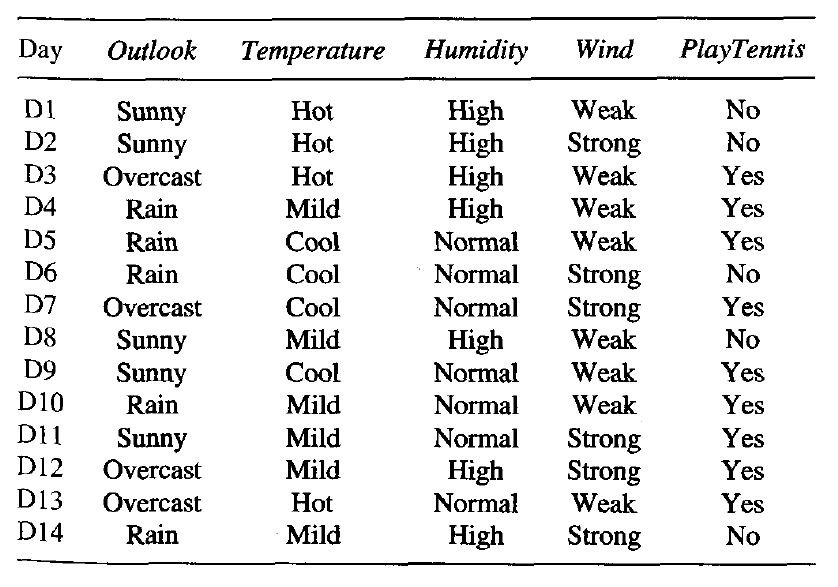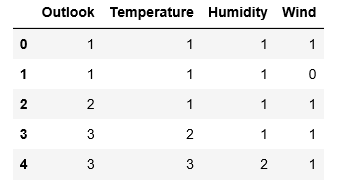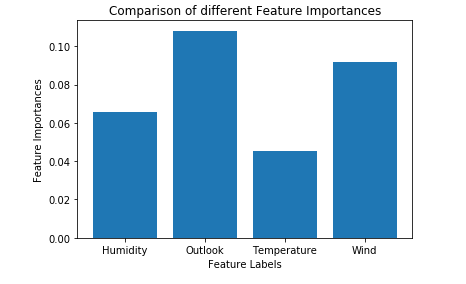# ML | Extra Tree Classifier for Feature Selection

Prerequisites: Decision Tree Classifier

Extremely Randomized Trees Classifier(Extra Trees Classifier) is a type of ensemble learning technique which aggregates the results of multiple de-correlated decision trees collected in a “forest” to output it’s classification result. In concept, it is very similar to a Random Forest Classifier and only differs from it in the manner of construction of the decision trees in the forest.

Each Decision Tree in the Extra Trees Forest is constructed from the original training sample. Then, at each test node, Each tree is provided with a random sample of k features from the feature-set from which each decision tree must select the best feature to split the data based on some mathematical criteria (typically the Gini Index). This random sample of features leads to the creation of multiple de-correlated decision trees.

To perform feature selection using the above forest structure, during the construction of the forest, for each feature, the normalized total reduction in the mathematical criteria used in the decision of feature of split (Gini Index if the Gini Index is used in the construction of the forest) is computed. This value is called the Gini Importance of the feature. To perform feature selection, each feature is ordered in descending order according to the Gini Importance of each feature and the user selects the top k features according to his/her choice.

Consider the following data:-Let us build a hypothetical Extra Trees Forest for the above data with five decision trees and the value of k which decides the number of features in a random sample of features be two. Here the decision criteria used will be Information Gain. First, we calculate the entropy of the data. Note the formula for calculating the entropy is:-where c is the number of unique class labels andis the proportion of rows with output label is i.

Therefore for the given data, the entropy is:-Let the decision trees be constructed such that:-

• 1st Decision Tree gets data with the features Outlook and Temperature:

Note that the formula for Information Gain is:-Thus,Similarly:• 2nd Decision Tree gets data with the features Temperature and Wind:

Using the above-given formulas:-• strong>3rd Decision Tree gets data with the features Outlook and Humidity:• 4th Decision Tree gets data with the features Temperature and Humidity:• 5th Decision Tree gets data with the features Wind and Humidity:Computing total Info Gain for each feature:-


Total Info Gain for Outlook     =     0.246+0.246   = 0.492

Total Info Gain for Temperature = 0.029+0.029+0.029 = 0.087

Total Info Gain for Humidity    = 0.151+0.151+0.151 = 0.453

Total Info Gain for Wind        =     0.048+0.048   = 0.096


• Thus the most important variable to determine the output label according to the above constructed Extra Trees Forest is the feature “Outlook”.

The below given code will demonstrate how to do feature selection by using Extra Trees Classifiers.

Step 1: Importing the required libraries

 import pandas as pd  import numpy as np  import matplotlib.pyplot as plt  from sklearn.ensemble import ExtraTreesClassifier

 # Changing the working location to the location of the file  cd C:\Users\Dev\Desktop\Kaggle     # Loading the data  df = pd.read_csv('data.csv')     # Separating the dependent and independent variables  y = df['Play Tennis']  X = df.drop('Play Tennis', axis = 1)     X.head()Step 3: Building the Extra Trees Forest and computing the individual feature importances

 # Building the model  extra_tree_forest = ExtraTreesClassifier(n_estimators = 5,                                          criterion ='entropy', max_features = 2)     # Training the model  extra_tree_forest.fit(X, y)     # Computing the importance of each feature  feature_importance = extra_tree_forest.feature_importances_     # Normalizing the individual importances  feature_importance_normalized = np.std([tree.feature_importances_ for tree in                                          extra_tree_forest.estimators_],                                          axis = 0)

Step 4: Visualizing and Comparing the results

 # Plotting a Bar Graph to compare the models  plt.bar(X.columns, feature_importance_normalized)  plt.xlabel('Feature Labels')  plt.ylabel('Feature Importances')  plt.title('Comparison of different Feature Importances')  plt.show()Thus the above-given output validates our theory about feature selection using Extra Trees Classifier. The importance of features might have different values because of the random nature of feature samples.

My Personal Notes arrow_drop_upCheck out this Author's contributed articles.

If you like GeeksforGeeks and would like to contribute, you can also write an article using contribute.geeksforgeeks.org or mail your article to contribute@geeksforgeeks.org. See your article appearing on the GeeksforGeeks main page and help other Geeks.

Please Improve this article if you find anything incorrect by clicking on the "Improve Article" button below.

Improved By : nidhi_biet

Article Tags :
Practice Tags :

2

Please write to us at contribute@geeksforgeeks.org to report any issue with the above content.